# Code

The code for the function is as shown below:

``````import os
import pandas as pd
import numpy as np
from datetime import datetime

def extract_data(directory_path, columns_to_extract, date_columns=[], rows_to_skip=0, output_filename='output.csv'):
"""
Extracts data from all Excel and CSV files in the specified directory and its subdirectories that contain all the
specified columns.

:param directory_path: The path to the directory to search for Excel and CSV files.
:param columns_to_extract: A list of column names to extract from each file.
:param date_columns: A list of column names to parse as dates using pd.to_datetime().
:param output_filename: The path and filename to save the extracted data in a csv
:return: A DataFrame containing the extracted data from all Excel and CSV files that contain the specified columns,
or None if no files contain the specified columns.
"""
start_time = datetime.now()
extracted_data = pd.DataFrame()
files_with_no_columns = []
extracted_columns = columns_to_extract.copy()
extracted_columns.extend(['File Name','SubDir','CreatedDate','LastModifiedDate'])

for root, dirs, files in os.walk(directory_path):
for file in files:
print('Started reading ' + file + ' at: {}'.format(datetime.now()) + ' ...')
if file.endswith('.xlsx') or file.endswith('.xls') or file.endswith('.csv'):
file_path = os.path.join(root, file)
columns_found_outer = False
if file.endswith('.csv'):
for col in columns_to_extract:
if col in df.columns:
columns_found_outer = True
#print(col + 'in csv true loop')
else:
columns_found_outer = False
#print(col + 'in false loop')
break
else:
for key in dfs.keys():
columns_found = False
for col in columns_to_extract:
if col in dfs[key].columns:
columns_found = True
#print(col + 'in true loop')
else:
columns_found = False
#print(col + 'in false loop')
break
if columns_found:
columns_found_outer = True
df = dfs[key]
break
if columns_found_outer:
if len(date_columns) > 0:
for col in date_columns:
if col in df.columns:
df[col] = pd.to_datetime(df[col])
df['File Name'] = file
df['SubDir'] = os.path.basename(root)

createx = modx = os.path.getctime(file_path)
xcreate = datetime.fromtimestamp(modx)
df['CreatedDate'] = xcreate

modx = os.path.getmtime(file_path)
xmod = datetime.fromtimestamp(modx)
df['LastModifiedDate'] = xmod
extracted_data = pd.concat([extracted_data, df[extracted_columns]], ignore_index=True)
#print(xmod)
if file.endswith('.csv'):
print(file + ' has been read')
else:
print(file + ' has been read and it was last modified on ' + xmod.strftime('%Y-%m-%d') + '. The name of the sheet that was read is: ' + sheet_read)
else:
files_with_no_columns.append(file_path)
if len(files_with_no_columns) > 0:
print("The following files do not contain the specified columns:")
for file_path in files_with_no_columns:
print(file_path)
if not extracted_data.empty:
extracted_data = extracted_data.applymap(lambda s: s.upper() if type(s) == str else s).fillna('')
extracted_data.to_csv(output_filename)
print('Started at: {}'.format(start_time) + '. \nEnded at: {}'.format(datetime.now()) + '. \nTime elapsed (hh:mm:ss.ms) {}'.format(datetime.now() - start_time))
return extracted_data
else:
print("Specified columns do not exist in any file in the provided directory.")
print('Started at: {}'.format(start_time) + '. \nEnded at: {}'.format(datetime.now()) + '. \nTime elapsed (hh:mm:ss.ms) {}'.format(datetime.now() - start_time))
return None
``````

# Explanation

1. The function `extract_data` takes in the following parameters:

• directory_path: the path to the directory to search for Excel and CSV files
• columns_to_extract: a list of column names to extract from each file
• date_columns: a list of column names to parse as dates using pd.to_datetime()
• rows_to_skip: the number of rows to skip when reading each file
• output_filename: the path and filename to save the extracted data in a CSV file
2. The function starts by creating an empty DataFrame called `extracted_data`.

3. It also initializes variables `files_with_no_columns` and `sheet_read` to track files that do not contain the specified columns and to keep track of the current sheet being read when extracting data from Excel files.

4. The list `extracted_columns` is created by copying the input `columns_to_extract` list and adding additional columns for the `filename`, `subdirectory name`, `file creation date`, and `last modified date`.

5. The function then loops through all the files in the specified directory and its subdirectories using os.walk().

6. For each file, it checks if it has a “.xlsx”, “.xls”, or “.csv” extension.

7. If the file is a CSV file, the function reads it into a DataFrame using `pd.read_csv()`, skipping the number of rows specified by `rows_to_skip`.

8. It then checks if all the columns in `columns_to_extract` are present in the DataFrame. If so, it sets `columns_found_outer` to `True` and proceeds to the next step. If not, it sets `columns_found_outer` to `False` and moves on to the next file.

9. If the file is an Excel file, the function reads all sheets in the file into a dictionary of DataFrames using `pd.read_excel()` and `sheet_name=None`. It then loops through all the sheets and all the columns in `columns_to_extract`, checking if each column is present in each sheet’s DataFrame. If all the columns are present in a sheet, it sets `columns_found` to `True` and proceeds to the next step. If not, it sets `columns_found` to `False` and moves on to the next sheet in the same Excel file. If at least one sheet contains all the specified columns, the function combines the DataFrames of all sheets into one using `pd.concat()`.

10. If `columns_found_outer` is `True`, it extracts the `filename`, `subdirectory name`, `file creation date`, and `last modified date` using `os.path.basename()`, `os.path.getctime()`, and `os.path.getmtime()`, and adds them as new columns to the DataFrame. It then appends the DataFrame to the `extracted_data` DataFrame.

11. If `columns_found_outer` is `False`, it increments the `files_with_no_columns` counter and prints a warning message.

12. Finally, the function checks if any files contained the specified columns. If not, it returns None. Otherwise, it sorts the `extracted_data` DataFrame by filename and saves it to a CSV file using `to_csv()`. It then prints the number of files processed, the number of files that did not contain the specified columns, and the path to the output file.

# Sample Usage

The function can be called in python as shown below:

``````# set directory path
directory_path = './Work' # Path for the directory where all the files containing data for  extraction are to be searched

# set the columns you want to extract
columns_to_extract = ['Region','Country', 'Product Number','Quantity','Date of Sale']
date_col = ['Date of Sale']
# set the rows to skip
skiprows = 0 #this basically will be number of rows in begining of the files which must be skipped to reach the header row of the data
output_filename = 'Regional Sales Data.csv'
#Call function
df = extract_data(directory_path,columns_to_extract,date_col,skiprows,output_filename)
``````

Now, assuming there were 12 separate files for past 12 months inside the folder then so long as all those files, irrespective of whether they are csv or excel, have the columns `Region`,`Country`, `Product Number`,`Quantity`,`Date of Sale`; the function will read the files and extract the data and return it to the dataframe `df`.

# GUI Implementation

A very basic GUI implementation of above function using PySimpleGUI with all codeis available here

## Usage

The script can directly be copied to a Jupyter cell or can be run from terminal. Following command should ensure all dependencies are installed:

``````py -m pip install pandas, numpy, pysimplegui
``````

Some things the GUI takes care of are:

• Allows selection of columns to be extracted from a sample `.csv` file
• Allows user to specify which of the selected columns should be parsed as `date`
• Gives a date based filename to `output`
• Shows colour coded log for which files were read in green and which were ignored in red background.

## Screenshots

Some screenshots of the resulting app are as shown below:

### Empty Form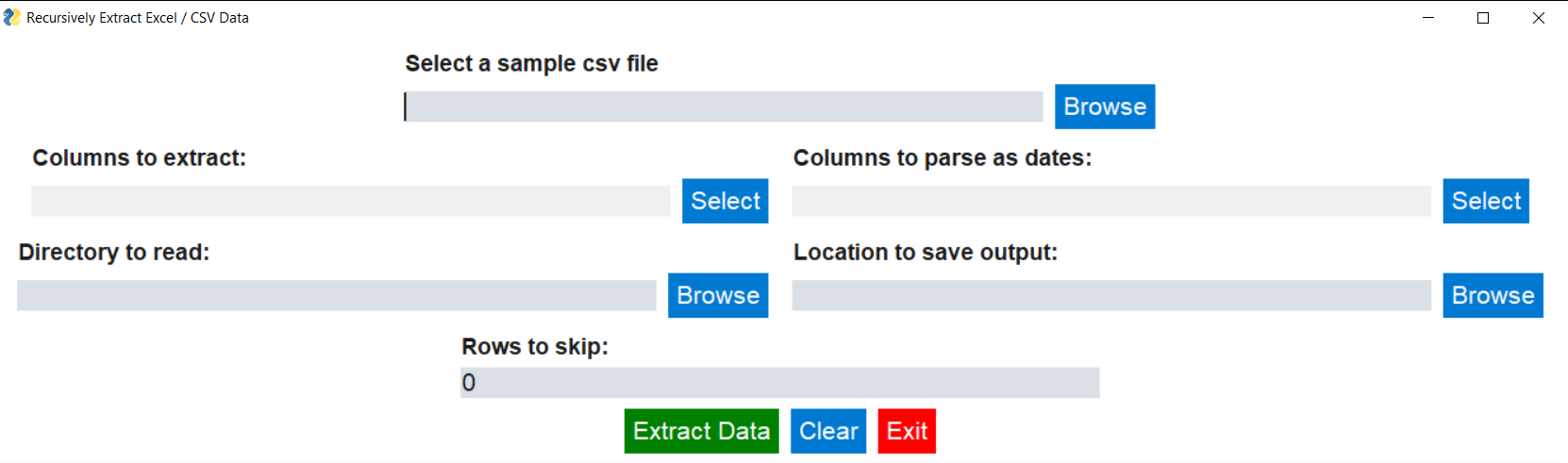### Filled Form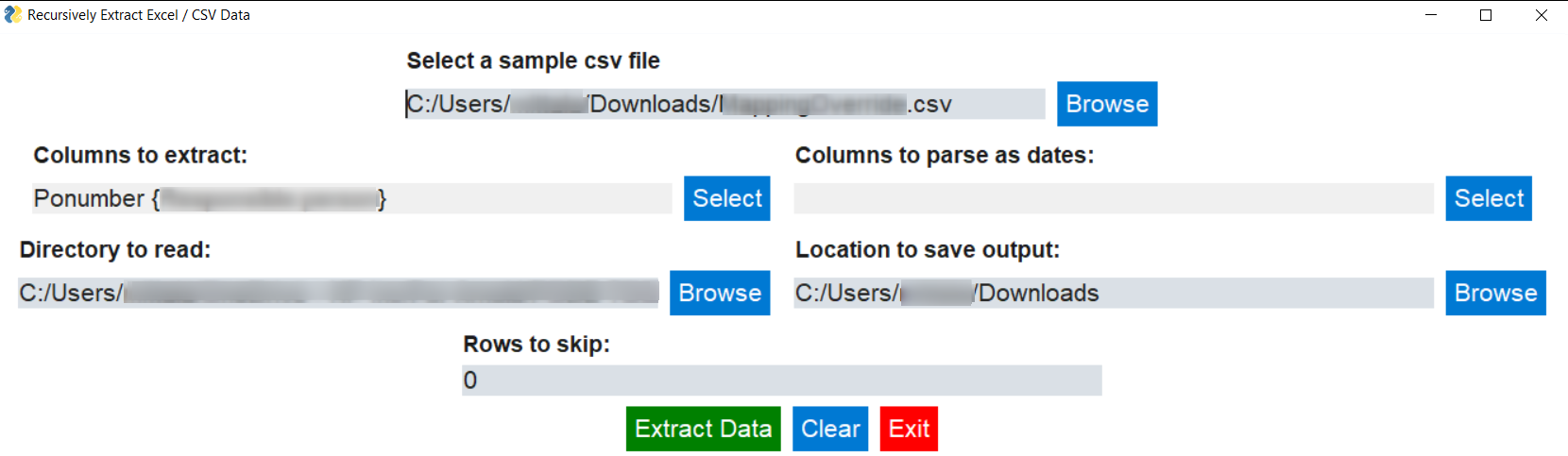### Displays extracted output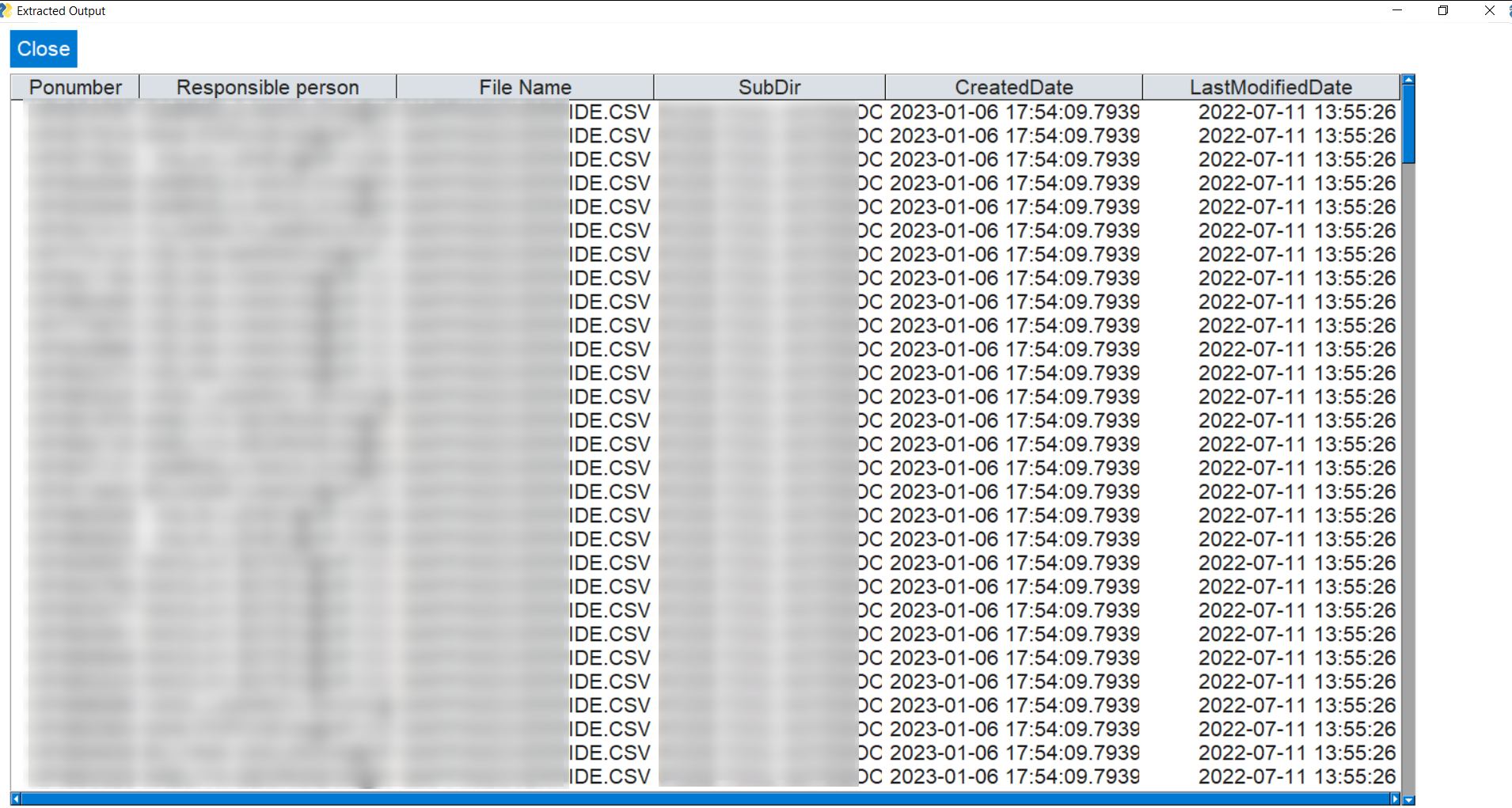### Displays filename of the output and location where it is saved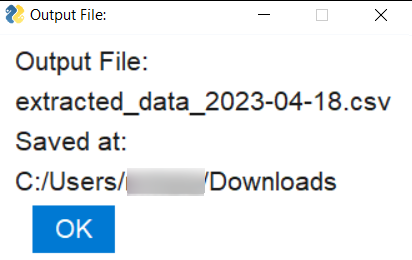### Colour coded log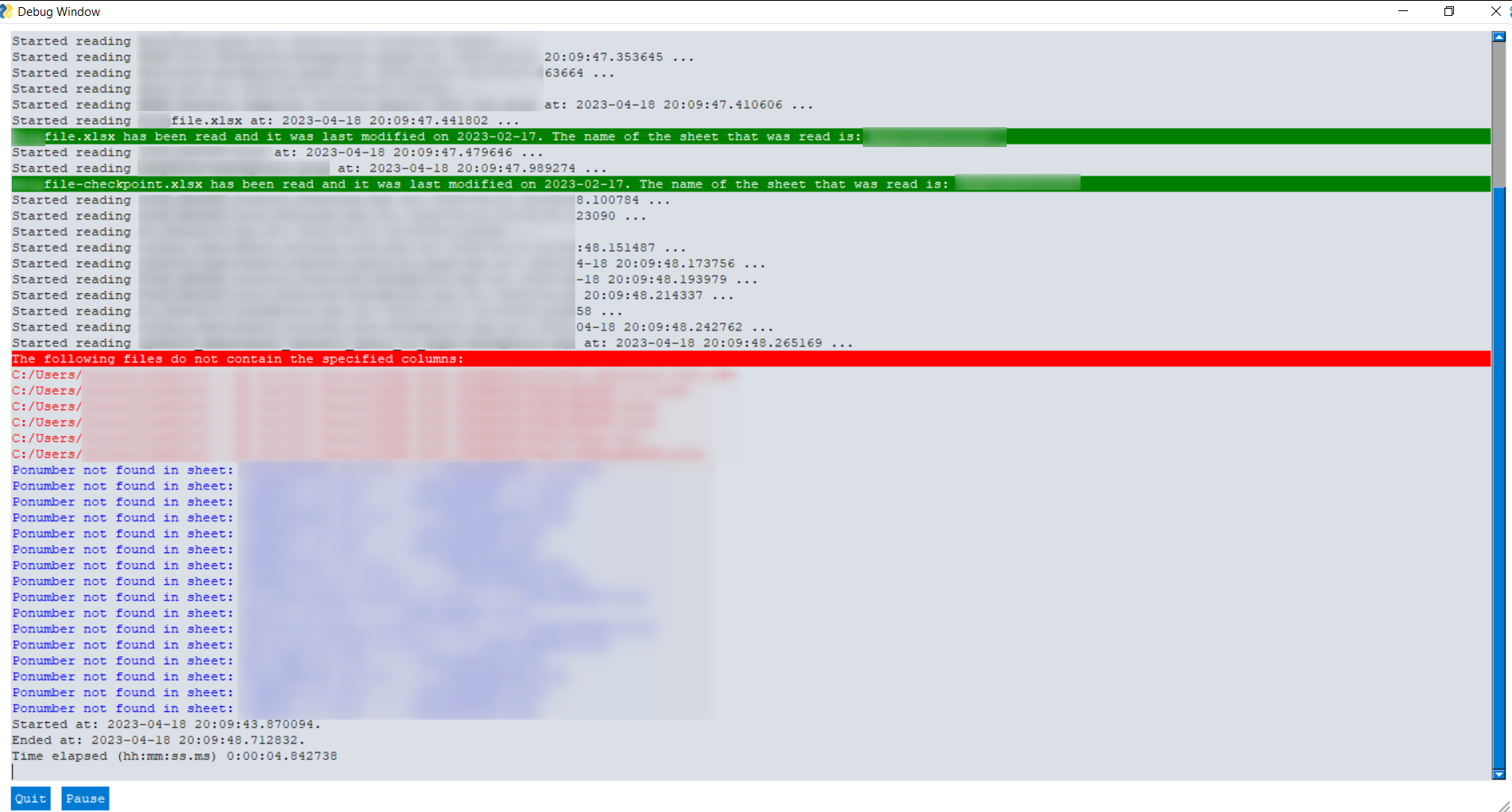Updated: